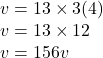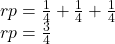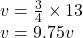Question

Find the voltage of the battery in the circuit above if R1 = 4 ohms, R2 = 4 ohms, R3 = 4 ohms and the current moving through the circuit is 13 A.

1.you didn’t say if its a parallel or a series circuit

Explanation:

If it’s a series circuit

V =IRHowever in parallel

calculate the Rp which will beso now you can say V=IR